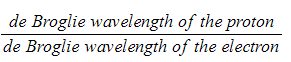# AQA A Level Physics复习笔记2.5.4 The de Broglie Wavelength

### The de Broglie Wavelength

• Using ideas based upon the quantum theory and Einstein’s theory of relativity, de Broglie suggested that the momentum (p) of a particle and its associated wavelength (λ) are related by the equation:• Since momentum p = mv, the de Broglie wavelength can be related to the speed of a moving particle (v) by the equation:• Since kinetic energy E = ½ mv2
• Momentum and kinetic energy can be related by:• Combining this with the de Broglie equation gives a form which relates the de Broglie wavelength of a particle to its kinetic energy:• Where:
• λ = the de Broglie wavelength (m)
• h = Planck’s constant (J s)
• p = momentum of the particle (kg m s-1)
• E = kinetic energy of the particle (J)
• m = mass of the particle (kg)
• v = speed of the particle (m s-1)

#### Worked Example

A proton and an electron are each accelerated from rest through the same potential difference.

Determine the ratio: